Updating search results...

# 11 Results

View
Selected filters:
• MCCRS.Math.Content.4.NBT.A.2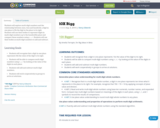Conditional Remix & Share Permitted
CC BY-NC-SA
Rating
0.0 stars

Students will explore multi-digit numbers and the relationship between ones, tens and hundreds; a digit in one place is 10x the digit in the place to its right. Students will use their bodies to represent digits in multi-digit numbers up to the hundredths place and compare these numbers using <, =, >. Students will use their bodies as multi-digit numbers to add and subtract.

Subject:
Mathematics
Material Type:
Activity/Lab
Teaching/Learning Strategy
08/08/2019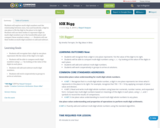Conditional Remix & Share Permitted
CC BY-SA
Rating
0.0 stars

Students will explore multi-digit numbers and the relationship between ones, tens and hundreds; a digit in one place is 10x the digit in the place to its right. Students will use their bodies to represent digits in multi-digit numbers up to the hundredths place and compare these numbers using <, =, >. Students will use their bodies as multi-digit numbers to add and subtract.

Subject:
Mathematics
Material Type:
Activity/Lab
Teaching/Learning Strategy
08/08/2019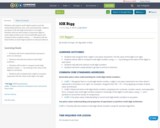Conditional Remix & Share Permitted
CC BY-NC-SA
Rating
0.0 stars

Students will explore multi-digit numbers and the relationship between ones, tens and hundreds; a digit in one place is 10x the digit in the place to its right. Students will use their bodies to represent digits in multi-digit numbers up to the hundredths place and compare these numbers using <, =, >. Students will use their bodies as multi-digit numbers to add and subtract.

Subject:
Mathematics
Material Type:
Activity/Lab
Teaching/Learning Strategy
08/08/2019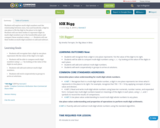Conditional Remix & Share Permitted
CC BY-NC-SA
Rating
0.0 stars

Students will explore multi-digit numbers and the relationship between ones, tens and hundreds; a digit in one place is 10x the digit in the place to its right. Students will use their bodies to represent digits in multi-digit numbers up to the hundredths place and compare these numbers using <, =, >. Students will use their bodies as multi-digit numbers to add and subtract.

Subject:
Mathematics
Material Type:
Activity/Lab
Teaching/Learning Strategy
08/08/2019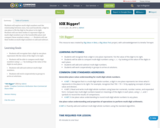Conditional Remix & Share Permitted
CC BY-NC-SA
Rating
0.0 stars

Students will explore multi-digit numbers and the relationship between ones, tens and hundreds; a digit in one place is 10x the digit in the place to its right. Students will use their bodies to represent digits in multi-digit numbers up to the hundredths place and compare these numbers using <, =, >. Students will use their bodies as multi-digit numbers to add and subtract.

Subject:
Mathematics
Material Type:
Activity/Lab
Teaching/Learning Strategy
01/28/2016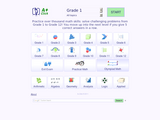Conditional Remix & Share Permitted
CC BY-NC-SA
Rating
0.0 stars

A+ Click is an interactive collection of more than 3700 math problems and answers for K-1 K-12 school program. It defines the personal level of math knowledge. You move up into the next level if you give 5 correct answers in a row. Practice makes perfect.

Subject:
Algebra
Geometry
Mathematics
Material Type:
Activity/Lab
Assessment
Game
Lecture Notes
Provider:
NSDL Staff
Provider Set:
Mathematics Gateways and Resources
Author:
Igor Kokcharov
02/16/2011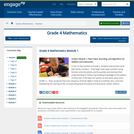Conditional Remix & Share Permitted
CC BY-NC-SA
Rating
0.0 stars

In this 25-day module of Grade 4, students extend their work with whole numbers.  They begin with large numbers using familiar units (hundreds and thousands) and develop their understanding of millions by building knowledge of the pattern of times ten in the base ten system on the place value chart (4.NBT.1).  They recognize that each sequence of three digits is read as hundreds, tens, and ones followed by the naming of the corresponding base thousand unit (thousand, million, billion).

Subject:
Mathematics
Numbers and Operations
Material Type:
Module
Provider:
New York State Education Department
Provider Set:
EngageNY
05/11/2013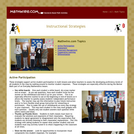Rating
0.0 stars

These strategies support active student participation in math lessons and allow teachers to assess the developing proficiency levels of all students in the class by walking around to monitor student responses. These strategies are especially effective during the Mental Math part of an Everyday Mathematics lesson.

Material Type:
Teaching/Learning Strategy
Provider:
Mathwire
Author:
Terry Kawas
02/16/2011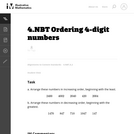Unrestricted Use
CC BY
Rating
0.0 stars

In this task students must place 4-digit numbers into order from smallest to greatest and greatest to smallest.

Subject:
Mathematics
Numbers and Operations
Material Type:
Activity/Lab
Provider:
Illustrative Mathematics
Provider Set:
Illustrative Mathematics
Author:
Illustrative Mathematics
05/01/2012Conditional Remix & Share Permitted
CC BY-NC-SA
Rating
0.0 stars

This resource is to be used by the teacher in a whole group instructional setting while teaching Place Value in a 4th grade classroom. This particular lesson is about writing numbers in standard and expanded form. There are links for student interaction using other websites at the end of the lesson.

Subject:
Numbers and Operations
Material Type:
Lesson
Lesson Plan
Author:
Jessica Brinker
10/27/2022Unrestricted Use
CC BY
Rating
0.0 stars

سيستكشف الطلاب الأعداد المتعددة الأرقام والعلاقة بين الآحاد والعشرات والمئات؛ الرقم في منزلة معينة يكون عشر مرات أكبر من قيمته إذا وجد في المنزلة التي عن يمينه. سيستخدم الطلاب أجسادهم لتمثيل الأرقام في الأعداد المتعددة الأرقام حتى منزلة المئات.
وسيقارنون تلك الأعداد باستخدام الرموز < ، = ، >. سيستخدم الطلاب أجسادهم لتمثيل عمليات جمع الأعداد وطرحها.

Subject:
Mathematics
Material Type:
Activity/Lab
Teaching/Learning Strategy